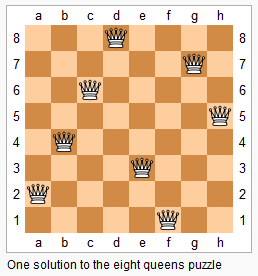• N皇后问题值在N*N的棋盘上要摆N个皇后，要求任何两个皇后不同行、不同列， 也不在同一条直线上。给定一个整数N，要求返回n皇后的摆法有多少种。 n=1，共1种， n=2，3共0种，n=8共92种。 def num1(n): if n < 1: ...
N皇后问题值在N*N的棋盘上要摆N个皇后，要求任何两个皇后不同行、不同列， 也不在同一条直线上。给定一个整数N，要求返回n皇后的摆法有多少种。 n=1，共1种， n=2，3共0种，n=8共92种。
def num1(n):
if n < 1:
return 0
record = [-1] * n	 #用来记录第k行的皇后放在了第几列，后边行的要避开这一列和斜线
return process1(0, record, n) 	#前0-1行已放好，从第0行开始放共多少种

def process1(i, record, n):
if i == n: 	#前n-1行已经放好，因为从0开始的，那么所有都放好了，完成一种放法
return 1
res = 0
for j in range(n): 	#遍历第i行的n列，看哪一列可以放
if isValid(record, i, j):  	#第i行第j列可以放，那么在record 中纪录，并计算i行放好后的i+1行开始的放法
record[i] = j
res += process1(i+1, record, n)
return res
def isValid(record, i, j):
for k in range(i):    #前i-1个放好了，逐个看是否限制第i行第j列
if record[k] == j or abs(record[k] - j) == abs(k - i):
return False
return True
print(num1(8))

展开全文• 局部搜索求解n皇后问题 python 代码： import random import time import copy import sys import numpy as np num=10000000 coll = [*2]*(num*2) coll = np.array(coll) def count_conflicts(q,n,i,j,c): ...


局部搜索求解n皇后问题 python

代码：

import random
import time
import copy
import sys
import numpy as np
num=10000000
coll = [*2]*(num*2)
coll = np.array(coll)
def count_conflicts(q,n,i,j,c):
conflict=c
#删除原有冲突
coll[q[i]+i]-=1
if(coll[q[i]+i]>0):
conflict-=1
coll[q[i]-i+n-1]-=1
if(coll[q[i]-i+n-1]>0):
conflict-=1
coll[q[j]+j]-=1
if(coll[q[j]+j]>0):
conflict-=1
coll[q[j]-j+n-1]-=1
if(coll[q[j]-j+n-1]>0):
conflict-=1
#现有冲突
coll[q[i]+j]+=1
if(coll[q[i]+j]>1):
conflict+=1
coll[q[i]-j+n-1]+=1
if(coll[q[i]-j+n-1]>1):
conflict+=1
coll[q[j]+i]+=1
if(coll[q[j]+i]>1):
conflict+=1
coll[q[j]-i+n-1]+=1
if(coll[q[i]-j+n-1]>1):
conflict+=1
return conflict

def recover_conflicts(q,n,i,j):
coll[q[i]+i]+=1
coll[q[i]-i+n-1]+=1
coll[q[j]+j]+=1
coll[q[j]-j+n-1]+=1
coll[q[i]+j]-=1
coll[q[i]-j+n-1]-=1
coll[q[j]+i]-=1
coll[q[j]-i+n-1]-=1
def count_all_conflicts(q,n):
conflict=0
for i in range(n):
coll[q[i]+i]+=1
coll[q[i]-i+n-1]+=1
for i in range(n*2):
for j in range(0,2):
if coll[i][j]>1:
# print(i,j)
conflict+=coll[i][j]-1
return conflict
if __name__ == "__main__":
q=random.sample(range(0,num),num)
min_conflicts = count_all_conflicts(q,num)
start=time.time()
# print(coll)
while(min_conflicts>0):
# print(q)
print(min_conflicts)
if(min_conflicts==0):
break
flag=0
for i in range(num):
for j in range(i+1,num):
# conflicts_before = count_conflicts(q,num,i,j)
# q[i],q[j]=q[j],q[i]
conflicts = count_conflicts(q,num,i,j,min_conflicts)
# print(min_conflicts,q)
if(conflicts<min_conflicts):
q[i],q[j]=q[j],q[i]
min_conflicts = conflicts
flag=1
break
else:
recover_conflicts(q,num,i,j)
if(flag==1):
break
if(flag==0):
q=random.sample(range(0,num),num)
min_conflicts = count_all_conflicts(q,num)
print('queen:',q)
end=time.time()
print('time',end-start)

能跑百万级皇后问题。
展开全文算法
• leetcode 51.n皇后(python) n 皇后问题研究的是如何将 n 个皇后放置在 n×n 的棋盘上，并且使皇后彼此之间不能相互攻击。 上图为 8 皇后问题的一种解法。 给定一个整数 n，返回所有不同的 n 皇后问题的解决方案。 ...
leetcode 51.n皇后(python)
n 皇后问题研究的是如何将 n 个皇后放置在 n×n 的棋盘上，并且使皇后彼此之间不能相互攻击。上图为 8 皇后问题的一种解法。
给定一个整数 n，返回所有不同的 n 皇后问题的解决方案。
每一种解法包含一个明确的 n 皇后问题的棋子放置方案，该方案中 'Q' 和 '.' 分别代表了皇后和空位。
示例:
输入: 4
输出: [
[".Q..",  // 解法 1
"...Q",
"Q...",
"..Q."],

["..Q.",  // 解法 2
"Q...",
"...Q",
".Q.."]
]
解释: 4 皇后问题存在两个不同的解法。

方法- 回溯
class Solution(object):
def solveNQueens(self, n):
if n < 1: return []

self.result = []
self.cols = []
self.pie = []
self.na = []

self.dfs(n, 0, [])
return self.generate_result(n)

def dfs(self, n, row, cur_state):
if row >= n:
self.result.append(cur_state)
return

for col in range(n):
if col in self.cols or row + col in self.pie or row - col in self.na:
continue

self.cols.append(col)
self.pie.append(row + col)
self.na.append(row - col)

self.dfs(n, row + 1, cur_state + [col])

self.cols.remove(col)
self.pie.remove(row + col)
self.na.remove(row - col)

def generate_result(self, n):
board = []
for res in self.result:
for i in res:
board.append('.' * i + 'Q' + '.' * (n - i - 1))
return [board[i: i + n] for i in range(0, len(board), n)]

简洁写法
class Solution(object):
def solveNQueens(self, n):
def DFS(queens, xy_dif, xy_sum):
p = len(queens)
if p == n:
result.append(queens)
return None
for q in range(n):
if q not in queens and p-q not in xy_dif and p+q not in xy_sum:
DFS(queens + [q], xy_dif + [p - q], xy_sum + [p + q])

result = []
DFS([], [], [])
return [['.' * i + 'Q' + '.' * (n - i - 1) for i in sol] for sol in result]

展开全文leetcode
• 找了数据结构书上的n皇后问题照着用Python的语法翻译一下。。毕竟是从书上翻下来的，侵删了啦～ n = int(input()) lst = [0 for i in range(n+1)] count = 0 def check(x, y): for i in range(1, x+1): if lst[i] ...
看很多童鞋写的n皇后问题代码很强大，但是我看不懂… 找了数据结构书上的n皇后问题照着用Python的语法翻译一下。。毕竟是从书上翻下来的，侵删了啦～
n = int(input())
lst = [0 for i in range(n+1)]
count = 0
def check(x, y):
for i in range(1, x+1):
if lst[i] == y:
return False
if i+lst[i] == x+y:
return False
if i-lst[i] == x-y:
return False
return True
def dfs(row):
global count
if row == n+1:
count += 1
return
for i in range(1, n+1):
if check(row, i):
lst[row] = i
dfs(row+1)
lst[row] = 0   # # 回溯 避免占着那啥不那啥，这也是为什么要用0覆盖的原因
return count
print(dfs(1))


展开全文• N皇后问题n皇后问题是将n个皇后放置在n*n的棋盘上，皇后彼此之间不能相互攻击。给定一个整数n，返回所有不同的n皇后问题的解决方案。每个解决方案包含一个明确的n皇后放置布局，其中“Q”和“.”分别表示一个女王和...算法 lintcode
• LeetCode 0051. N-Queens N 皇后【Hard】【Python】【回溯】leetcode
• n 皇后问题研究的是如何将 n皇后放置在 n×n 的棋盘上，并且使皇后彼此之间不能相互攻击。 上图为 8 皇后问题的一种解法。 给定一个整数 n，返回所有不同的 n 皇后问题的解决方案。 每一种解法包含一个明确的 n ...dfs
• n皇后问题python语言实现） N皇后是回溯算法的经典问题之一。 请在一个n*n的正方形盘面上布置n名皇后（规则：每一名皇后都可以自上下左右斜方向攻击，所以要保证每一行、每一列、每一斜线上都只有一名皇后）。 ...算法
• 题目描述 n 皇后问题研究的是如何将 n 个皇后放置在 n×n 的棋盘上，并且使皇后彼此之间不能相互攻击。 上图为 8 皇后问题的一种...N皇后问题是一道典型的递归求解问题，常见为8皇后、4皇后问题。递归对象为在0−...递归
• def isValid(board, x, y): for i in range(x + 1): for j in range(len(board[i])): if board[i][j] and (j == y or abs(x - i) == abs(y - j)): ...def nQueenProblem(board, row, n, res): if ro算法
• ## N皇后问题（Python实现）

千次阅读 多人点赞 2019-05-27 17:01:41
n皇后问题研究的是如何将n个皇后放置在n×n的棋盘上，并且使皇后彼此之间不能相互攻击。 也就是说：存在一个N*N的棋盘，要放N个棋子，每个棋子不同行，不同列，不同（正反）对角线，如图： 解题思路： 递归 + 回朔...LeetCode
• n皇后问题 研究的是如何将 n个皇后放置在 n×n 的棋盘上，并且使皇后彼此之间不能相互攻击。 给你一个整数 n ，返回所有不同的n皇后问题 的解决方案。 每一种解法包含一个不同的n 皇后问题 的棋子放置方案，该方案...leetcode
• ## pythonN皇后问题

千次阅读 2019-08-15 21:08:03
需要将N皇后放在N*N的棋盘上，条件是任何一个皇后都不能威胁其他皇后，即任何两个皇后都不能吃掉对方。怎样才能做到这一点呢？已将这些皇后放在什么地方呢？ 思路： 1.以第一个和第二个皇后为例，第一个皇后默认...
• n 皇后问题研究的是如何将 n 个皇后放置在 n×n 的棋盘上，并且使皇后彼此之间不能相互攻击。 上图为 8 皇后问题的一种解法。 给定一个整数 n，返回 n 皇后不同的解决方案的数量。 示例: 输入: 4 输出: 2 ...
• 数据结构中常见的问题，最近复习到了，用python做一遍。 # 检测（x,y）这个位置是否合法（不会被其他皇后攻击到） def is_attack(queue, x, y): for i in range(x): if queue[i] == y or abs(x - i) == abs(queue...
• [Leetcode]N皇后 Leetcode-N皇后 题目描述 n 皇后问题研究的是如何将 n 个皇后放置在 n×n 的棋盘上，并且使皇后彼此之间不能相互攻击。 上图为 8 皇后问题的一种解法。 给定一个整数 n，返回所有不同的 n 皇后问题...leetcode
• 八皇后[n皇后] 问题表述为：在8×8格的国际象棋上摆放8个皇后，使其不能互相攻击，即任意两个皇后都不能处于同一行、同一列或同一斜线上，问有多少种摆法。答案是92种 可以看看遍历过程 方便理解 c = 0 def queen(A,...算法 递归法 数据结构 leetcode
• Python 递归方式解N皇后问题，解后绘制棋盘
• 51. N皇后 n 皇后问题研究的是如何将 n 个皇后放置在 n×n 的棋盘上，并且使皇后彼此之间不能相互攻击。 上图为 8 皇后问题的一种解法。 给定一个整数 n，返回所有不同的 n 皇后问题的解决方案。 每一...leetcode
• 首先，N皇后是一个非常经典的递归+回溯的问题。 然后，写一个判断函数check来判断某个点是不是可以放置的点是该算法的重点 为了防止内存爆炸，N皇后问题是用一个[1，n]的矩阵存储结果的。该矩阵的下标值是皇后所在...算法 递归算法
• 这位大佬的解决方法写得非常好，递归的代码非常简洁，我只是理解了然后进一步精简了一下代码 def queen(result, board, row=0): n = len(board) if row >= n: result.append(board) ... for col in range(n):
• LeetCode -------- 51.N皇后 N 皇后 n 皇后问题 研究的是如何将 n 个皇后放置在 n×n 的棋盘上，并且使皇后彼此之间不能相互攻击。 给你一个整数 n ，返回所有不同的 n 皇后问题 的解决方案。 每一种解法包含一个...
• 回溯算法：N皇后问题N皇后问题思路Python 基础解法C 基础解法Python 简化方法 N皇后问题 N皇后问题是个经典问题，在 N*N 的国际象棋棋盘内放下N个皇后有几种解法，使其不能互相攻击，属于典型的回溯问题。国际象棋中...算法 c++
• n 皇后问题研究的是如何将 n皇后放置在 n×n 的棋盘上，并且使皇后彼此之间不能相互攻击。 上图为 8 皇后问题的一种解法。 给定一个整数 n，返回所有不同的 n 皇后问题的解决方案。 每一种解法包含一个明确...
• 皇后问题python解法 递归解法，board 是一维列表，下标表示行，值表示皇后所在的列。 board = [-1] * 8 def printboard(result): for v in result: length = len(result) print('□ '*v + '■ ' + '□ '* ......

# n皇后问题pythonpython 订阅